# Basic Techniques: Pairs

Hey guys, welcome back to Sudokumania, the home of online sudoku where we provide you with free sudokus daily. Yes we do have daily sudokus and not just classic sudokus, but a whole lot of Sudoku Variants. So, first of all, apologies for the long absence.

Let's continue our series of Sudoku solving techniques that will not only help you improve as a Sudoku player but also improve your logical and problem solving skills. Today, let's have a look at another basic technique. Last post we spoke about using Direct and Hidden Singles, we will now have a look at how we can extend the concept of singles to pair of digits.

In today’s post we will have a look at the two techniques involving Pairs which are the next stage in the basic solving techniques. They are Naked Pairs (Direct Pairs) and Hidden Pairs. I will also place links to sudokus which use these techniques at the end of the post for you to practice the said techniques.

## Naked Pair

Naked Pairs is an extension of Naked Singles. In Naked Single we had only one digit possible in a cell. Naked Pairs is a technique used when we have only 2 digits possible in 2 cells which share a constraint; meaning the cells have to be in the same row, or column or 3x3 box. So when we have exactly 2 digits as possible candidates (pencil marks) in exactly two cells which share a constraint, then we can safely eliminate these 2 digits from all other cells within that constraint. Let’s have a look at the image below. It is only for explanatory purpose.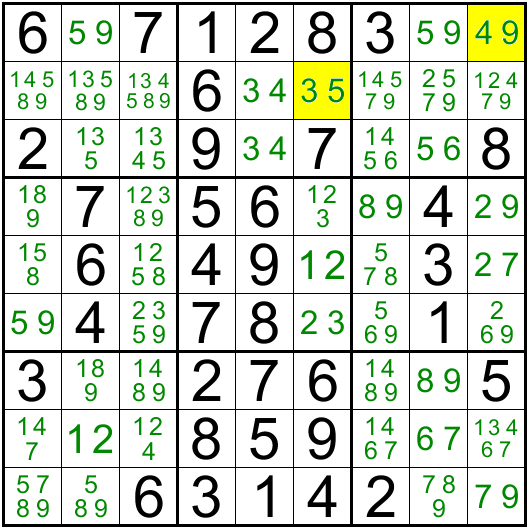In Box 2, we have 3 cells with pencil marks. The constraint here is the 3x3 box. The two cells R2C5 and R3C5 have the same two digits (3 and 4) as pencil marks, and this forms your Naked Pair. Which means we can remove both these digits from all other cells within that constraint (Box 2) so we can eliminate the digit 3 from R2C6 and we get a Naked Single 5. Similarly look at Row 1. We have two digits 5 and 9 as pencil marks in exactly 2 cells, R1C2 and R1C8. Which means we can eliminate 5 and 9 from all other cells within Row 1. So we get 4 in R1C9. This is known as a Naked Pair.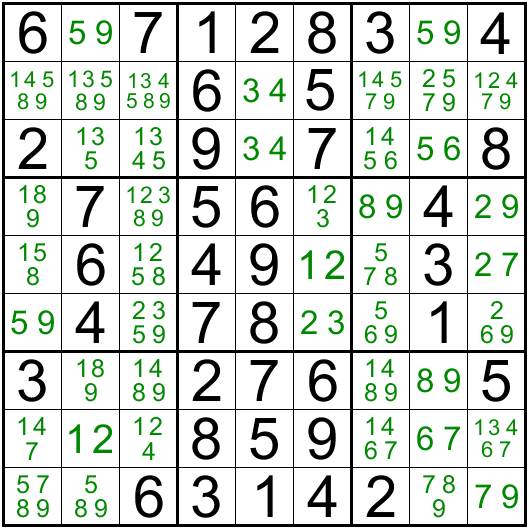## Hidden Pair

Just like the hidden single, a hidden pair is “hidden” among other possible candidates within a given constraint. To understand this better, let us take a look at the image below. Once again I would like to say that the below image is not a valid sudoku but only for demo purposes.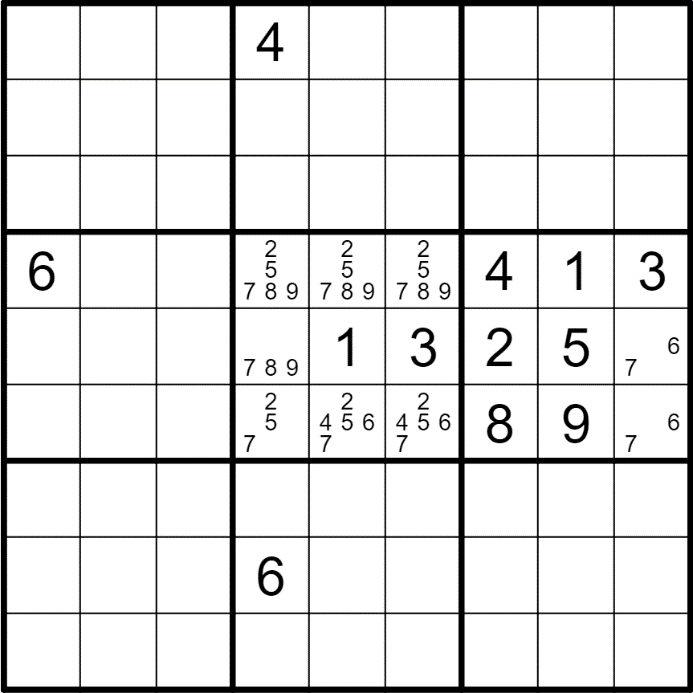Let’s have a look at box 5. All the cells have multiple digits possible in them. On closer observation, we see that the digits 4 and 6 can be placed only in the cells R6C5 or R6C6. Now we do not know the exact position of these digits. For example if R6C5 is a 4 then R6C6 will be a 6 and if R6C5 is a 6 then R6C6 will be a 4. So no matter what these two digits can occur only within these two cells R6C5 and R6C6. Hence we can eliminate all other possible digits from these two cells. Now we have converted the Hidden Pair into a Naked Pair as is seen in the picture below.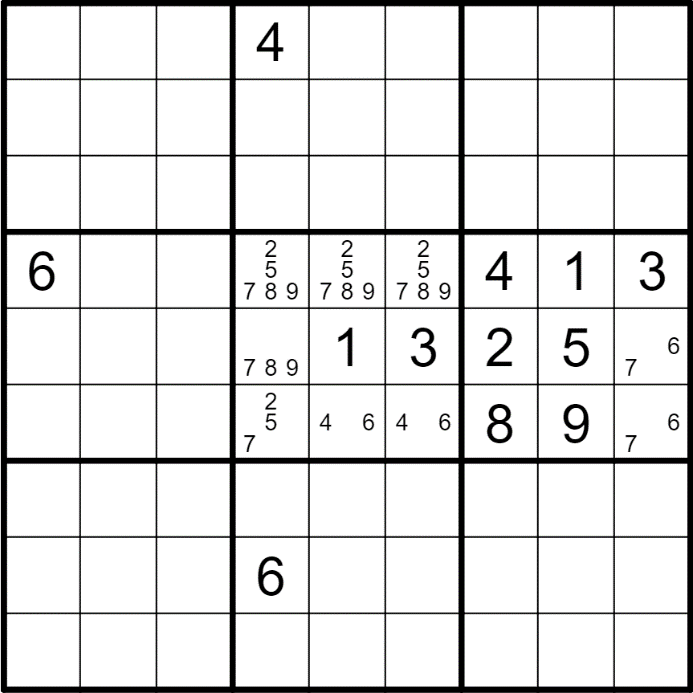Since these two cells share the box as well as the row, we can eliminate both these digits from Box 5 and Row 6, which allows us to place the 7 in R6C9 and subsequently 6 in R5C9.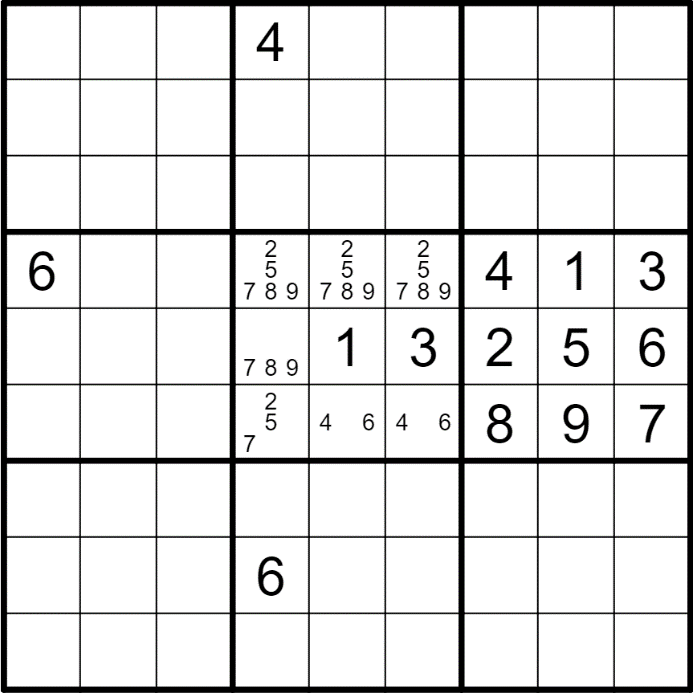All sudokus irrespective of the difficulty level, will begin with the use of these basic techniques. Do give it a try for yourself and you will see a marked difference in how you approach a sudoku to be solved. In the next post I will share how I normally begin solving a sudoku, before I put in more posts on solving techniques.

## Practice Sudoku

Put the concepts shared in today's post to practice by trying to solve this Classic Sudoku from 19th February, 2022. Until next time, keep practising!